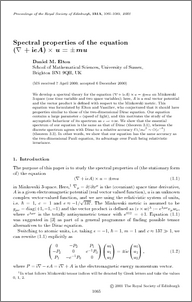# Spectral properties of the equation (' + ieA) ' u = '?

Elton, Daniel M. (2001) Spectral properties of the equation (' + ieA) ' u = '? Proceedings of the Royal Society of Edinburgh: Section A Mathematics, 131 (5). pp. 1065-1089. ISSN 1473-7124Preview
PDF (download.pdf)
Download (325Kb) | Preview

## Abstract

We develop a spectral theory for the equation ( + ieA) × u = ±mu on Minkowski 3-space (one time variable and two space variables); here, A is a real vector potential and the vector product is defined with respect to the Minkowski metric. This equation was formulated by Elton and Vassiliev, who conjectured that it should have properties similar to those of the two-dimensional Dirac equation. Our equation contains a large parameter c (speed of light), and this motivates the study of the asymptotic behaviour of its spectrum as c → +∞. We show that the essential spectrum of our equation is the same as that of Dirac (theorem 3.1), whereas the discrete spectrum agrees with Dirac to a relative accuracy δλ/mc2 ~ O(c−4) (theorem 3.3). In other words, we show that our equation has the same accuracy as the two-dimensional Pauli equation, its advantage over Pauli being relativistic invariance.

Item Type: Journal Article Proceedings of the Royal Society of Edinburgh: Section A Mathematics The final, definitive version of this article has been published in the Journal, Proceedings of the Royal Society of Edinburgh: Section A Mathematics, 131 (5), pp 1065-1089 2001, © 2001 Cambridge University Press. RAE_import_type : Journal article RAE_uoa_type : Pure Mathematics /dk/atira/pure/researchoutput/libraryofcongress/qa ?? QA MATHEMATICS ?? Faculty of Science and Technology > Mathematics and Statistics 2394 ep_importer 01 Apr 2008 09:18 Yes Published 29 Apr 2019 14:26 http://eprints.lancs.ac.uk/id/eprint/2394

### Actions (login required)

 View Item